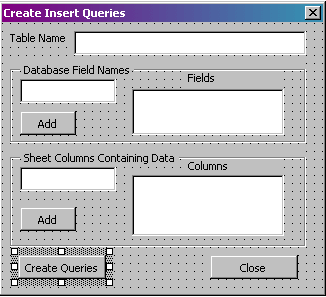## Tuesday, June 13, 2006

### Excel: Creating Static SQL Insert Statements Via Macro

There are times that I don’t particularly need to insert data from a spreadsheet right into a database, but would rather have a script generated or a series of SQL statements generated from spreadsheet data. I usually do this in cases where I have a set population sent to me via spreadsheet, and I am creating a script to initialize tables. This way, in the even of a crash or if I need to set up a QA/UA/Testing environment, I can simply run the script and have my initial tables and sample data ready to go. This works out great when I have a set population for an online report.

The easiest way to do this from Excel is using the debug.print statement, which will output text directly to the Immediate window. This has some drawbacks, since the immediate window can only hold so much data. If the script that is generated is large, I can output directly to a file, or into another sheet. Below is a sample macro that will generate the insert statements I need based on the sample form in Figure 1 and display the results into the immediate window. This can easily be modified if needed to output to a file or to another sheet inside of a workbook.Figure 1. The Form

Option Explicit

'Add if there is something in the textbox
If txtColumn.Text <> "" Then
End If

'Clear and set focus back to the textbox
txtColumn.Text = ""
txtColumn.SetFocus
End Sub

'Add if there is something in the textbox
If txtField.Text <> "" Then
End If

'Clear and set focus back to the textbox
txtField.Text = ""
txtField.SetFocus
End Sub

Private Sub cmdClose_Click()
'Close the form
End Sub

Private Sub cmdCreate_Click()
Dim sql_prefix, sql, sql_suffix As String
Dim LastRow, x, y As Long
Dim temp As Variant

'Get the number of cells. If there are 0, exit this function
If WorksheetFunction.CountA(Cells) > 0 Then
LastRow = Cells.Find(What:="*", After:=[A1], _
SearchOrder:=xlByRows, _
SearchDirection:=xlPrevious).Row
Else
MsgBox "There are no cells in this sheet!"
Exit Sub
End If

If lstCols.ListCount <> lstFields.ListCount Then
MsgBox "Field count does not match Column Count"
Exit Sub
End If

'Create out prefix and suffix
sql_suffix = ");"
sql_prefix = "insert into " & txtTable.Text & " ("

''Add the fields to the prefix
For x = 0 To (lstFields.ListCount - 1)
sql_prefix = sql_prefix & lstFields.List(x) & ","
Next

'Remove the extra comma and add the remainder of the prefix
sql_prefix = Left(sql_prefix, Len(sql_prefix) - 1)
sql_prefix = sql_prefix & ") values ("

'Now, create the actual queries to use for each row in the sheet
For x = 1 To LastRow
'Preset SQL to equal the prefix, clearing the value from the last loop
sql = sql_prefix

'For each column listed in the list box
For y = 0 To (lstCols.ListCount - 1)
sql = sql & "'" & Range(Trim(lstCols.List(y)) & Trim(Val(x))).Value & "',"
Next

'Remove the extra comma and append the suffix. Then print the results
'to the immediate window
sql = Left(sql, Len(sql) - 1)
sql = sql & sql_suffix
Debug.Print sql
Next
End Sub

Now, all I need to do is fill out the form, and the SQL statements will be generated for me. I can use this to generate my initial scripts based on a user population.

#### 1 comment:

Excel Statements said...

I am currently struggling try to create a solution which only draws a fraction of a string from a vendors cookie. Is this possible along these methods?